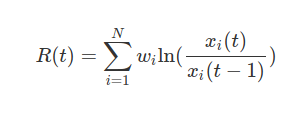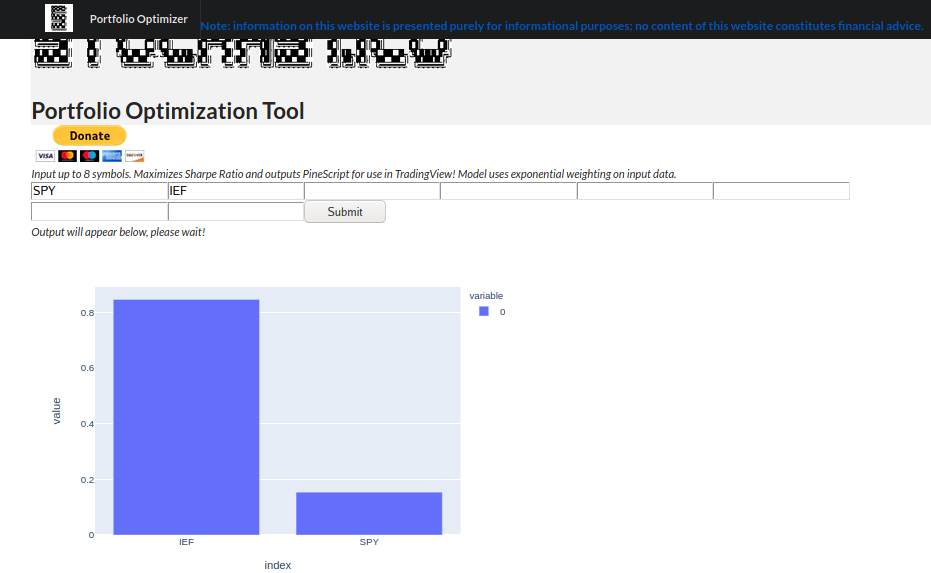# Creating and Visualizing a Portfolio with STOCHAST.ICU and TradingViewR(t), being the total weighted returns at time t, time being positive integers.Expected Return= 0.09429448127450267, Volatility= 0.04612413333354159, Sharpe= 1.6107507264635263. Data fit over 6988 days.

Statistician, MMath Statistics Student at the University of Waterloo

## More from Aaron Janeiro Stone

Statistician, MMath Statistics Student at the University of Waterloo